Games
Problems
Go Pro!

# Arithmetic

Quizzes > Mathematics > Arithmetic

# Featured Quizzes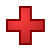Practice your addition facts, adding single digit numbers. Students who remember these facts do their work much more efficiently than students who...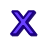Practice your multiplication tables with this quiz. Students who remember their multiplication facts work much more efficiently than students who...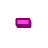Practice subtraction facts to help improve your ability to solve problems quickly, and without relying on a calculator.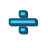Practice your division facts using this simple quiz. Students who remember their division and multiplication facts complete their work more...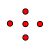If you see a picture containing some dots, can you count how many there are, and then find the correct number in a list of choices? This quiz is...Practice your multiplication facts with this simple math quiz at The Problem Site.Practice your math facts with this simple math quiz at The Problem Site.Practice your multiplication facts with this simple math quiz at The Problem Site.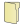# Full Directory Listing

CountingLike us on Facebook to get updates about new resources